# Clustering Distance Measures Hierarchical Clustering k Means Algorithms

• Slides: 53Clustering Distance Measures Hierarchical Clustering k -Means Algorithms 1The Problem of Clustering u. Given a set of points, with a notion of distance between points, group the points into some number of clusters, so that members of a cluster are in some sense as nearby as possible. 2Example x x x x xx x x x x x x 3The Curse of Dimensionality u. While clustering looks intuitive in 2 dimensions, many applications involve 10 or 10, 000 dimensions. u. High-dimensional spaces look different: the probability of random points being close drops quickly as the dimensionality grows. 4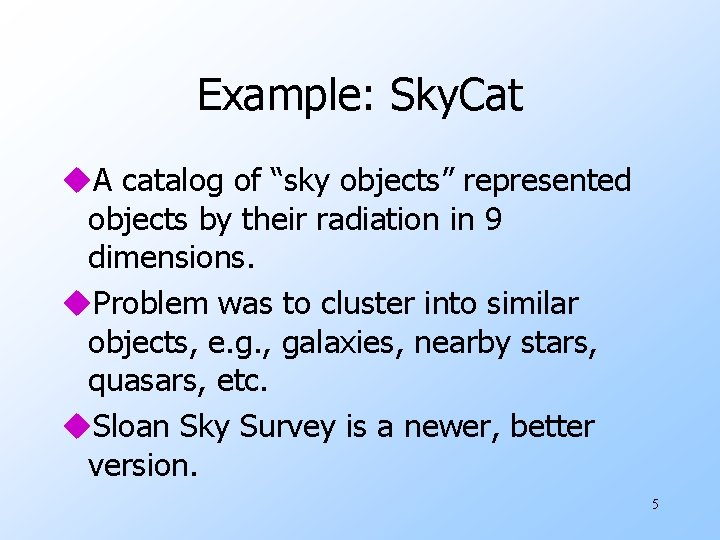Example: Sky. Cat u. A catalog of “sky objects” represented objects by their radiation in 9 dimensions. u. Problem was to cluster into similar objects, e. g. , galaxies, nearby stars, quasars, etc. u. Sloan Sky Survey is a newer, better version. 5Example: Clustering CD’s u. Intuitively: music divides into categories, and customers prefer a few categories. w But what are categories really? u. Represent a CD by the customers who bought it. u. Similar CD’s have similar sets of customers, and vice-versa. 6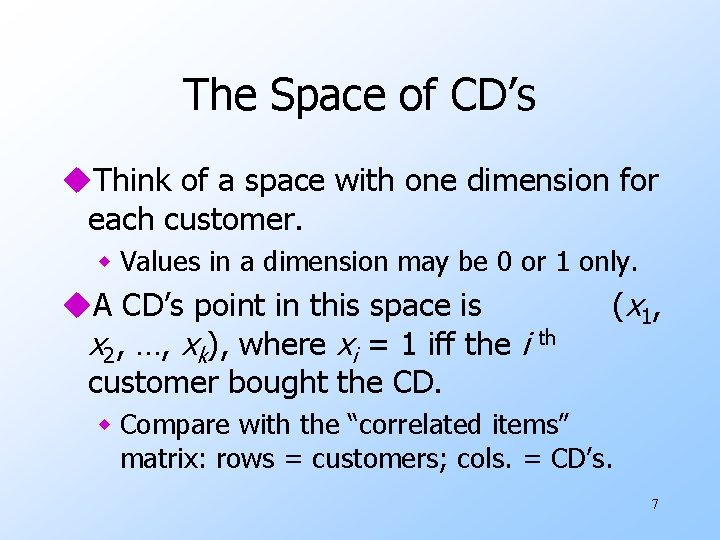The Space of CD’s u. Think of a space with one dimension for each customer. w Values in a dimension may be 0 or 1 only. u. A CD’s point in this space is x 2, …, xk), where xi = 1 iff the i customer bought the CD. th ( x 1 , w Compare with the “correlated items” matrix: rows = customers; cols. = CD’s. 7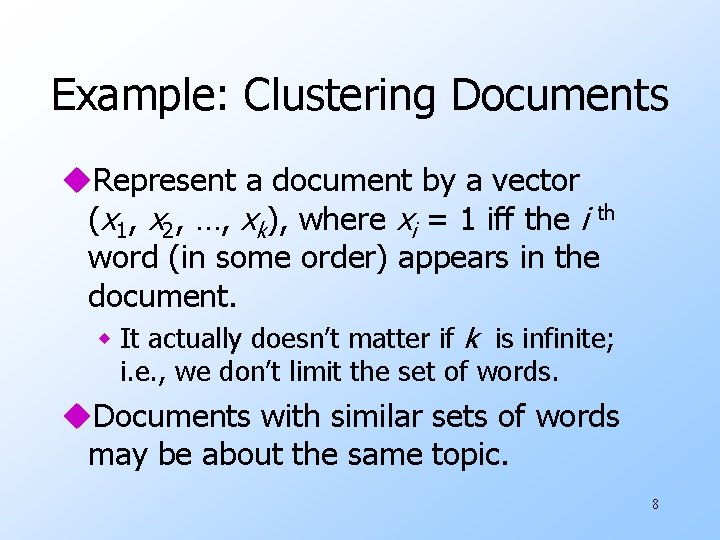Example: Clustering Documents u. Represent a document by a vector (x 1, x 2, …, xk), where xi = 1 iff the i th word (in some order) appears in the document. w It actually doesn’t matter if k is infinite; i. e. , we don’t limit the set of words. u. Documents with similar sets of words may be about the same topic. 8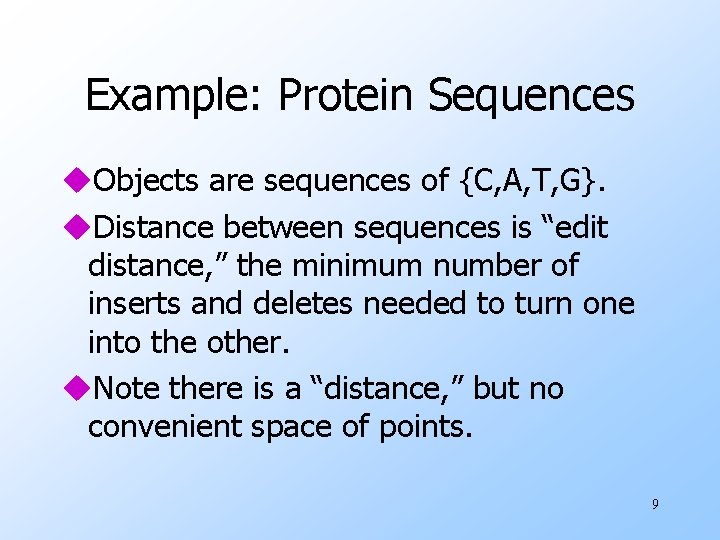Example: Protein Sequences u. Objects are sequences of {C, A, T, G}. u. Distance between sequences is “edit distance, ” the minimum number of inserts and deletes needed to turn one into the other. u. Note there is a “distance, ” but no convenient space of points. 9Distance Measures u Each clustering problem is based on some kind of “distance” between points. u Two major classes of distance measure: 1. Euclidean : based on position of points in some k -dimensional space. 2. Noneuclidean : not. 10Axioms of a Distance Measure u d is a distance measure if it is a function from pairs of points to reals such that: 1. 2. 3. 4. d(x, x) = 0. d(x, y) = d(y, x). d(x, y) > 0. d(x, y) < d(x, z) + d(z, y) (triangle inequality). 11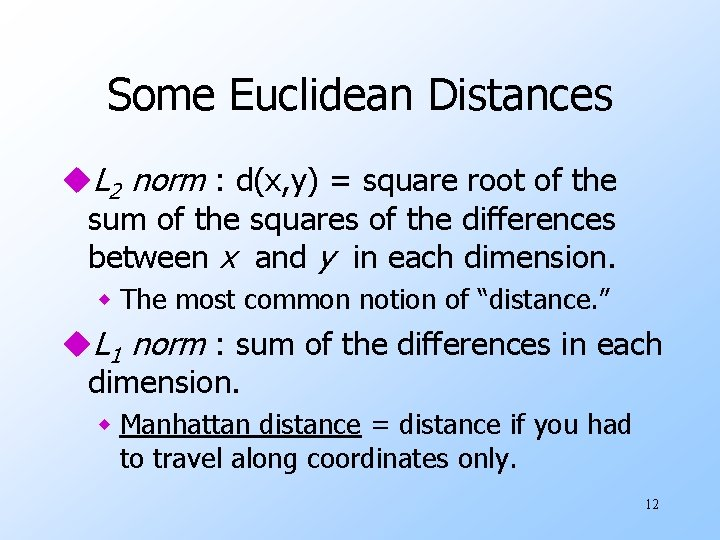Some Euclidean Distances u. L 2 norm : d(x, y) = square root of the sum of the squares of the differences between x and y in each dimension. w The most common notion of “distance. ” u. L 1 norm : sum of the differences in each dimension. w Manhattan distance = distance if you had to travel along coordinates only. 12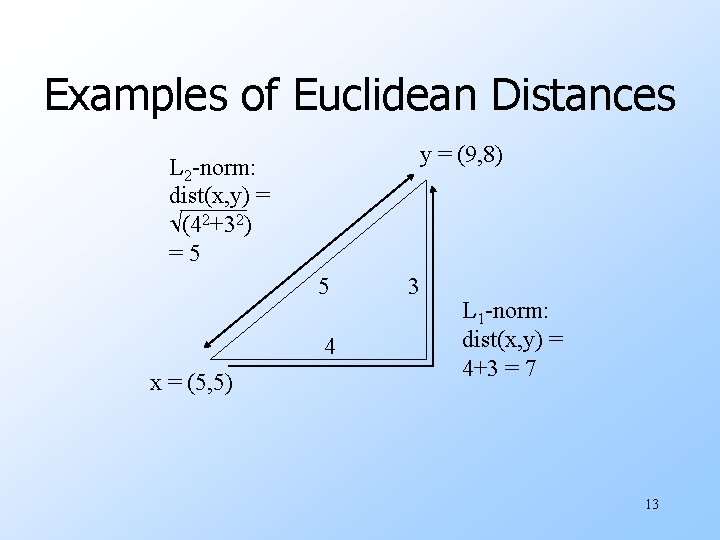Examples of Euclidean Distances y = (9, 8) L 2 -norm: dist(x, y) = (42+32) =5 5 4 x = (5, 5) 3 L 1 -norm: dist(x, y) = 4+3 = 7 13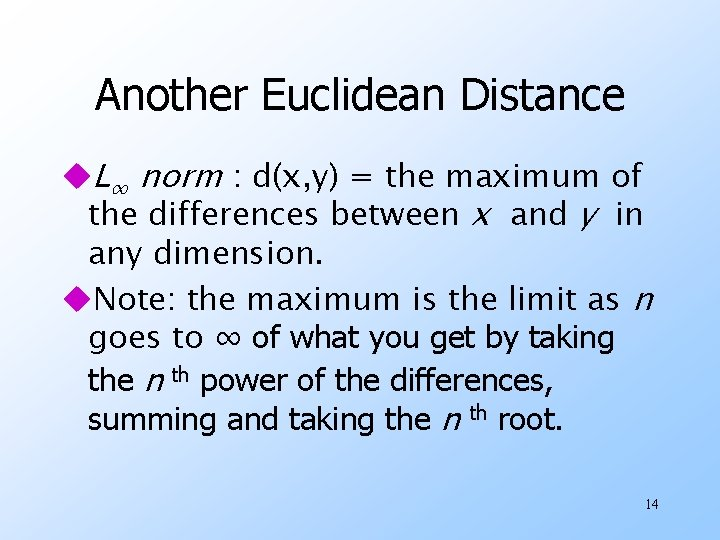Another Euclidean Distance u. L∞ norm : d(x, y) = the maximum of the differences between x and y in any dimension. u. Note: the maximum is the limit as n goes to ∞ of what you get by taking the n th power of the differences, summing and taking the n th root. 14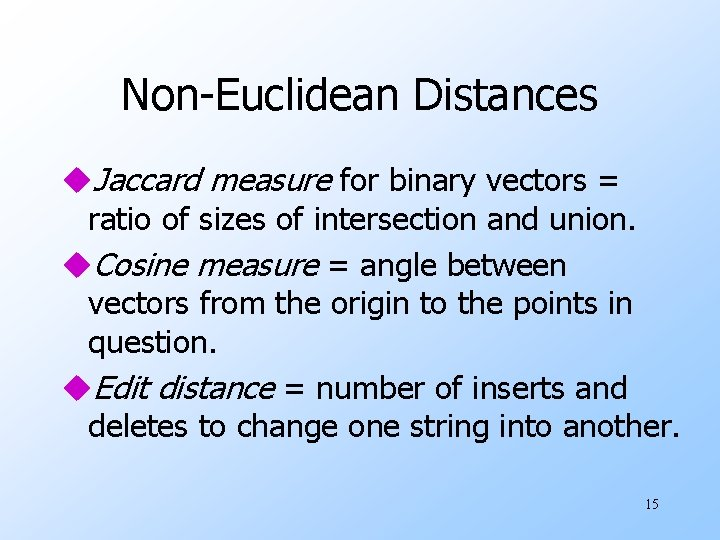Non-Euclidean Distances u. Jaccard measure for binary vectors = ratio of sizes of intersection and union. u. Cosine measure = angle between vectors from the origin to the points in question. u. Edit distance = number of inserts and deletes to change one string into another. 15Jaccard Measure u. Example: p 1 = 00111; p 2 = 10011. w Size of intersection = 2; size of union = 4, J. M. = 1/2. u. Need to make a distance function satisfying triangle inequality and other laws. ud(x, y) = 1 - J. M. works. 16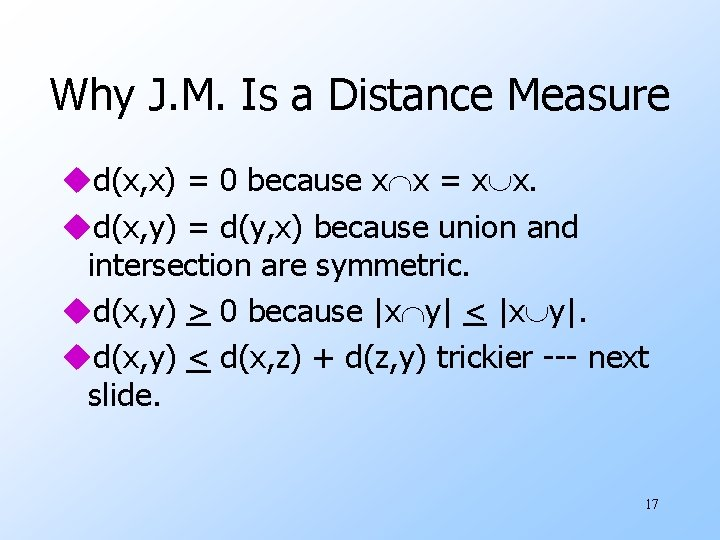Why J. M. Is a Distance Measure ud(x, x) = 0 because x x = x x. ud(x, y) = d(y, x) because union and intersection are symmetric. ud(x, y) > 0 because |x y| < |x y|. ud(x, y) < d(x, z) + d(z, y) trickier --- next slide. 17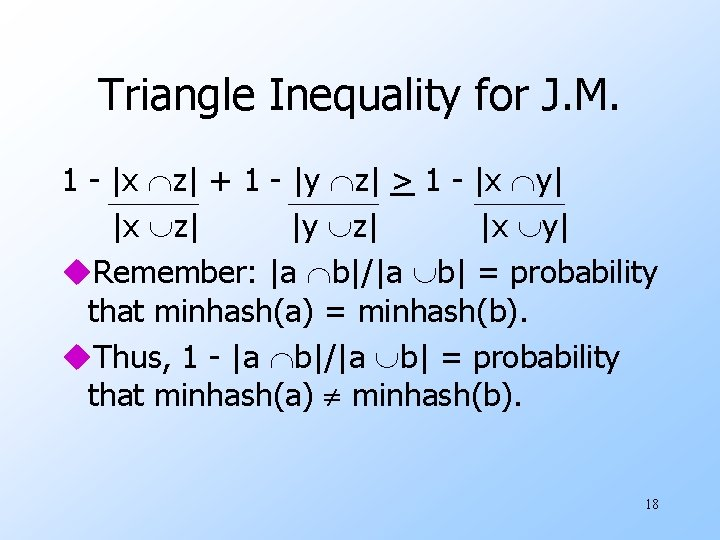Triangle Inequality for J. M. 1 - |x z| + 1 - |y z| > 1 - |x y| |x z| |y z| |x y| u. Remember: |a b|/|a b| = probability that minhash(a) = minhash(b). u. Thus, 1 - |a b|/|a b| = probability that minhash(a) minhash(b). 18Triangle Inequality --- (2) u. So we need to observe that prob[minhash(x) minhash(y)] < prob[minhash(x) minhash(z)] + prob[minhash(z) minhash(y)] u. Clincher: whenever minhash(x) minhash(y), one of minhash(x) minhash(z) and minhash(z) minhash(y) must be true. 19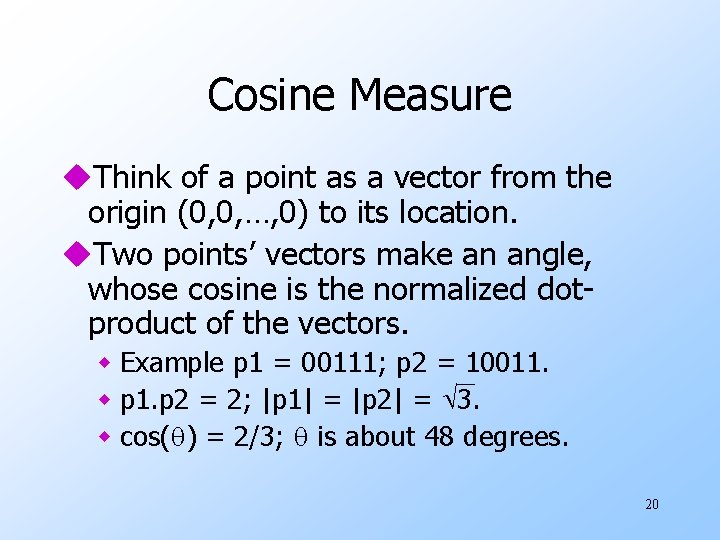Cosine Measure u. Think of a point as a vector from the origin (0, 0, …, 0) to its location. u. Two points’ vectors make an angle, whose cosine is the normalized dotproduct of the vectors. w Example p 1 = 00111; p 2 = 10011. w p 1. p 2 = 2; |p 1| = |p 2| = 3. w cos( ) = 2/3; is about 48 degrees. 20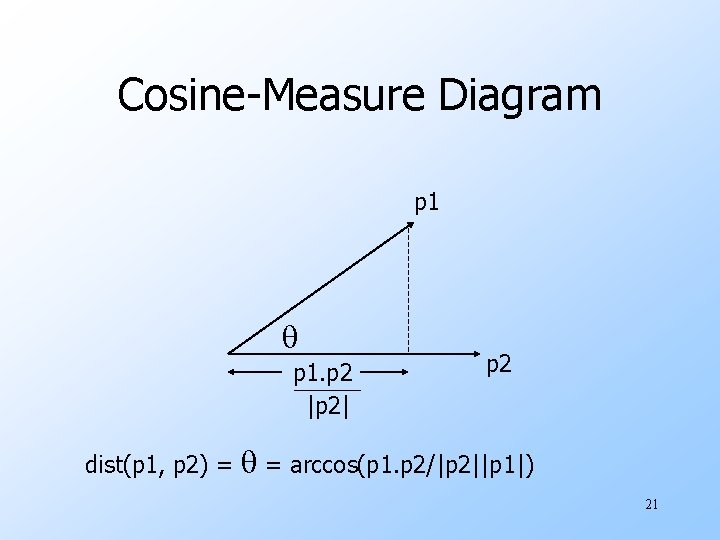Cosine-Measure Diagram p 1. p 2 |p 2| dist(p 1, p 2) = p 2 = arccos(p 1. p 2/|p 2||p 1|) 21Why? Dot product is invariant under rotation, so pick convenient coordinate system. p 1. p 2 = x 1*x 2. |p 2| = x 2. x 1 p 1 = (x 1, y 1) p 2 = (x 2, 0) x 1 = p 1. p 2/|p 2| 22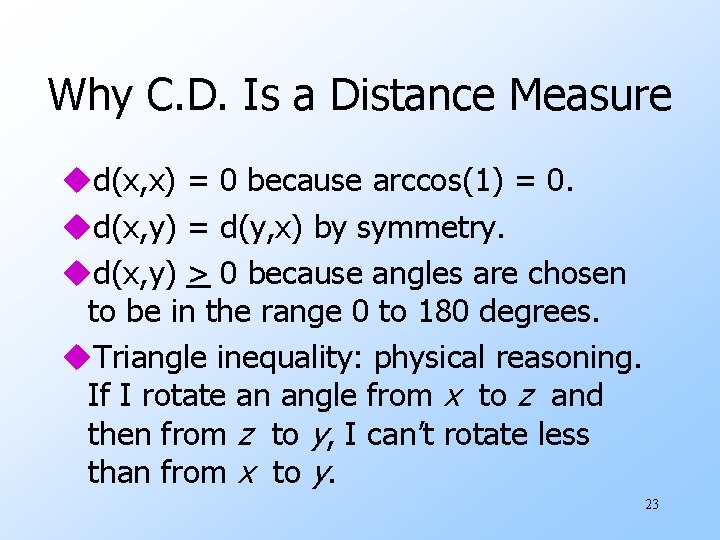Why C. D. Is a Distance Measure ud(x, x) = 0 because arccos(1) = 0. ud(x, y) = d(y, x) by symmetry. ud(x, y) > 0 because angles are chosen to be in the range 0 to 180 degrees. u. Triangle inequality: physical reasoning. If I rotate an angle from x to z and then from z to y, I can’t rotate less than from x to y. 23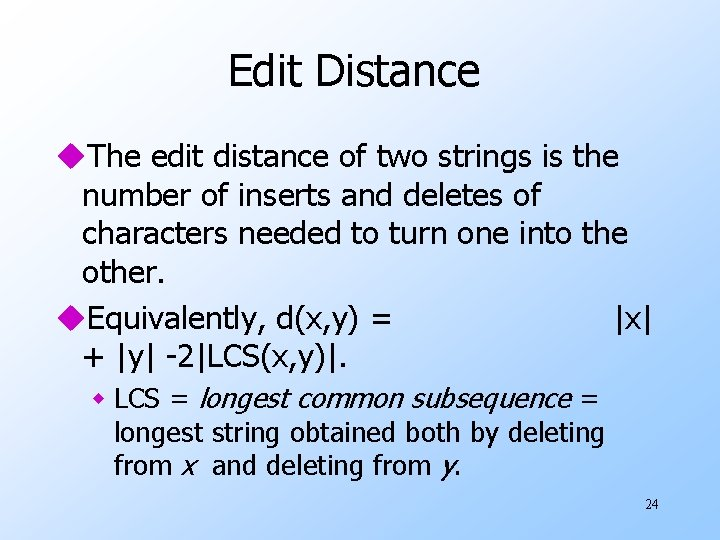Edit Distance u. The edit distance of two strings is the number of inserts and deletes of characters needed to turn one into the other. u. Equivalently, d(x, y) = |x| + |y| -2|LCS(x, y)|. w LCS = longest common subsequence = longest string obtained both by deleting from x and deleting from y. 24Example ux = abcde ; y = bcduve. u. Turn x into y by deleting a, then inserting u and v after d. w Edit-distance = 3. u. Or, LCS(x, y) = bcde. u|x| + |y| - 2|LCS(x, y)| = 5 + 6 – 2*4 = 3. 25Why E. D. Is a Distance Measure ud(x, x) = 0 because 0 edits suffice. ud(x, y) = d(y, x) because insert/delete are invertible. ud(x, y) > 0: no notion of negative edits. u. Triangle inequality: changing x to z and then to y is one way to change x to y. 26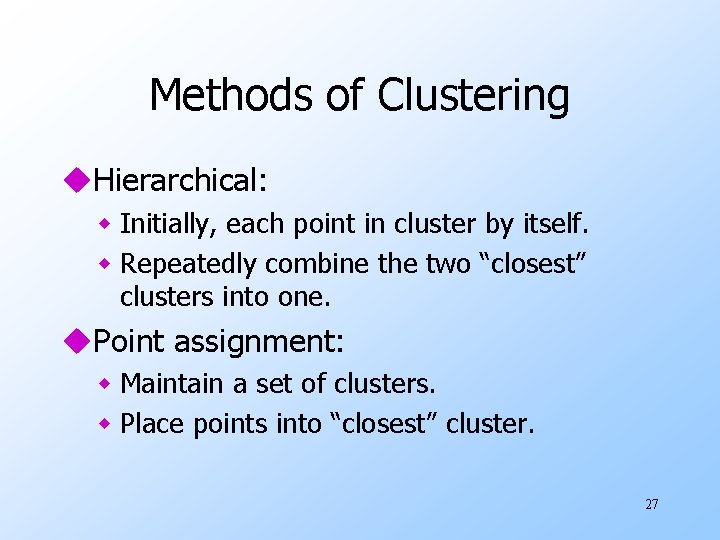Methods of Clustering u. Hierarchical: w Initially, each point in cluster by itself. w Repeatedly combine the two “closest” clusters into one. u. Point assignment: w Maintain a set of clusters. w Place points into “closest” cluster. 27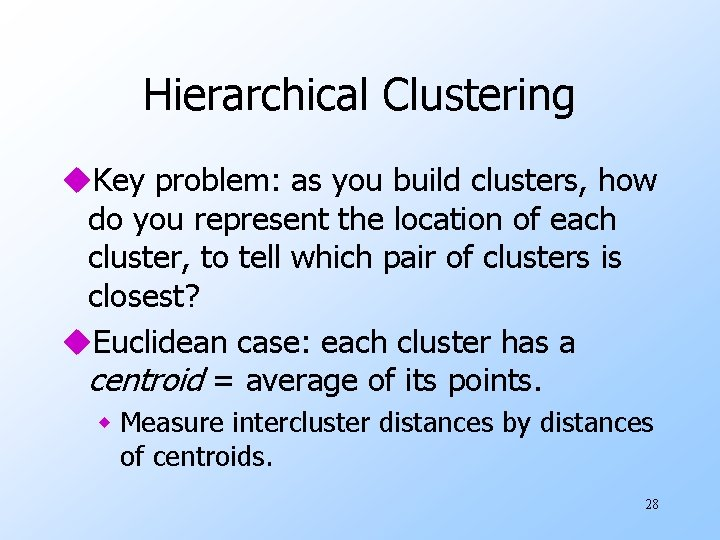Hierarchical Clustering u. Key problem: as you build clusters, how do you represent the location of each cluster, to tell which pair of clusters is closest? u. Euclidean case: each cluster has a centroid = average of its points. w Measure intercluster distances by distances of centroids. 28Example (5, 3) o (1, 2) o x (1. 5, 1. 5) x (1, 1) o (2, 1) o (0, 0) x (4. 7, 1. 3) o (4, 1) x (4. 5, 0. 5) o (5, 0) 29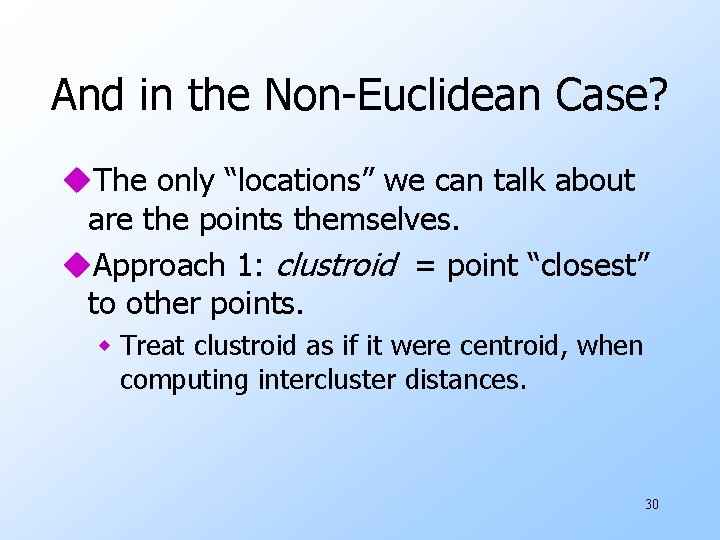And in the Non-Euclidean Case? u. The only “locations” we can talk about are the points themselves. u. Approach 1: clustroid = point “closest” to other points. w Treat clustroid as if it were centroid, when computing intercluster distances. 30Example clustroid 1 2 6 3 4 5 clustroid intercluster distance 31Other Approaches to Defining “Nearness” of Clusters u. Approach 2: intercluster distance = minimum of the distances between any two points, one from each cluster. u. Approach 3: Pick a notion of “cohesion” of clusters, e. g. , maximum distance from the clustroid. w Merge clusters whose union is most cohesive. 32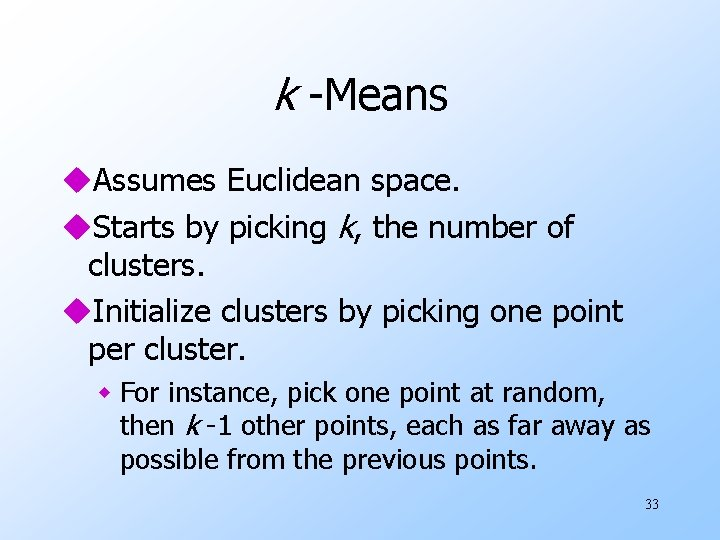k -Means u. Assumes Euclidean space. u. Starts by picking k, the number of clusters. u. Initialize clusters by picking one point per cluster. w For instance, pick one point at random, then k -1 other points, each as far away as possible from the previous points. 33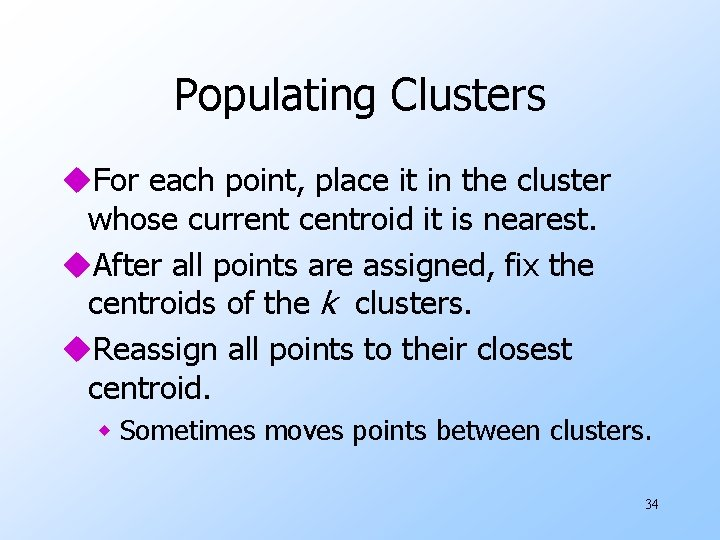Populating Clusters u. For each point, place it in the cluster whose current centroid it is nearest. u. After all points are assigned, fix the centroids of the k clusters. u. Reassign all points to their closest centroid. w Sometimes moves points between clusters. 34Example 2 Reassigned points 4 6 7 5 x 3 1 x 8 Clusters after first round 35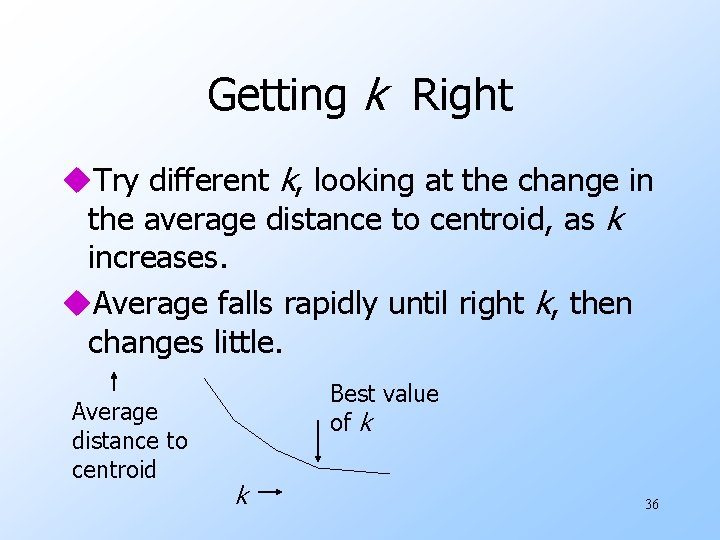Getting k Right u. Try different k, looking at the change in the average distance to centroid, as k increases. u. Average falls rapidly until right k, then changes little. Average distance to centroid Best value of k k 36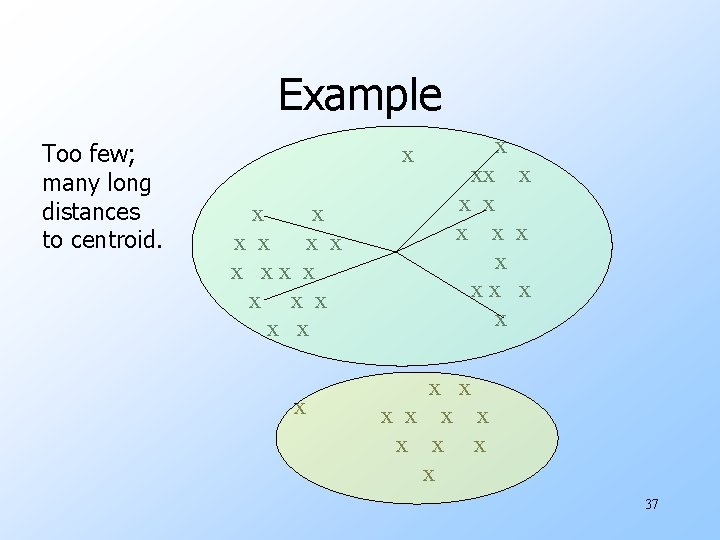Example Too few; many long distances to centroid. x x x x xx x x x x x x 37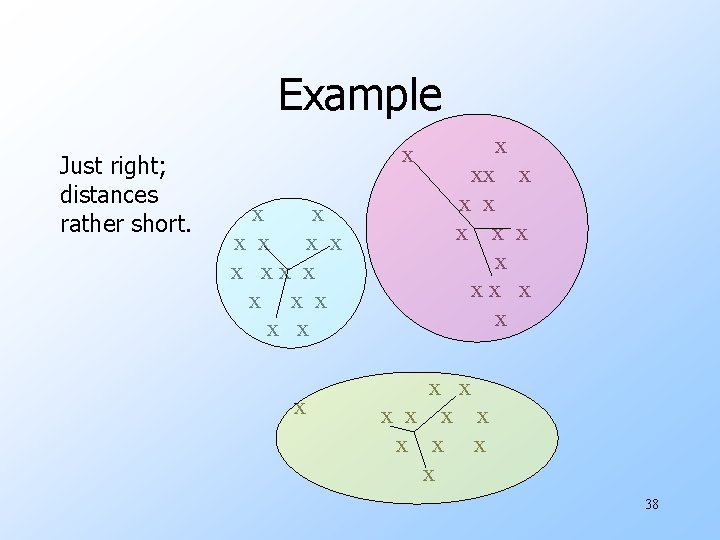Example Just right; distances rather short. x x x x xx x x x x x x 38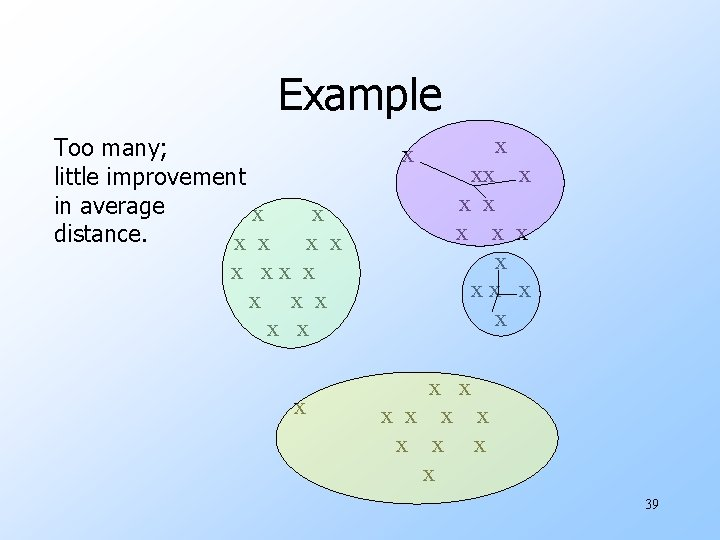Example Too many; little improvement in average x distance. x x x xx x xx x x x 39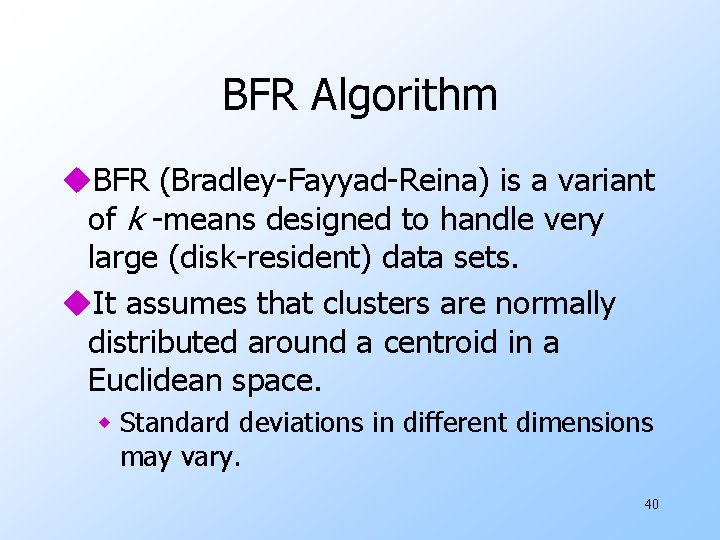BFR Algorithm u. BFR (Bradley-Fayyad-Reina) is a variant of k -means designed to handle very large (disk-resident) data sets. u. It assumes that clusters are normally distributed around a centroid in a Euclidean space. w Standard deviations in different dimensions may vary. 40BFR --- (2) u. Points are read one main-memory-full at a time. u. Most points from previous memory loads are summarized by simple statistics. u. To begin, from the initial load we select the initial k centroids by some sensible approach. 41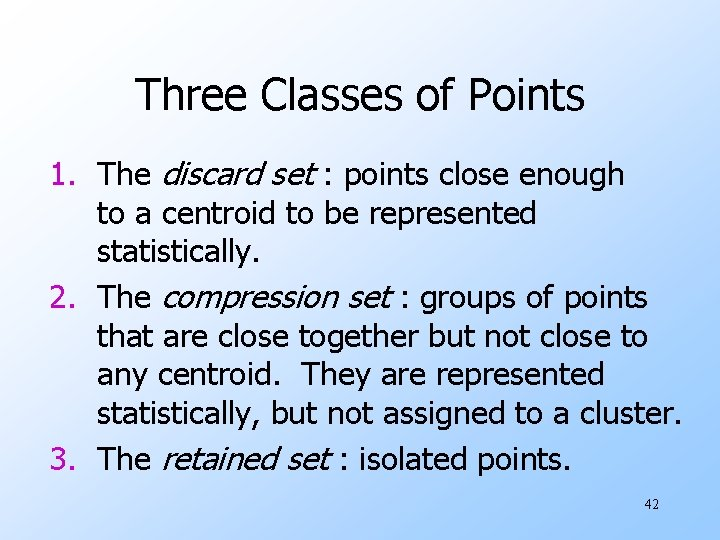Three Classes of Points 1. The discard set : points close enough to a centroid to be represented statistically. 2. The compression set : groups of points that are close together but not close to any centroid. They are represented statistically, but not assigned to a cluster. 3. The retained set : isolated points. 42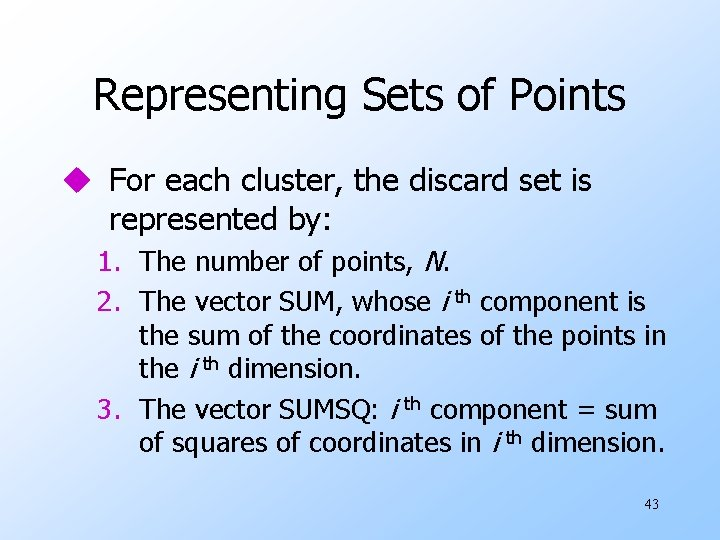Representing Sets of Points u For each cluster, the discard set is represented by: 1. The number of points, N. 2. The vector SUM, whose i th component is the sum of the coordinates of the points in the i th dimension. 3. The vector SUMSQ: i th component = sum of squares of coordinates in i th dimension. 43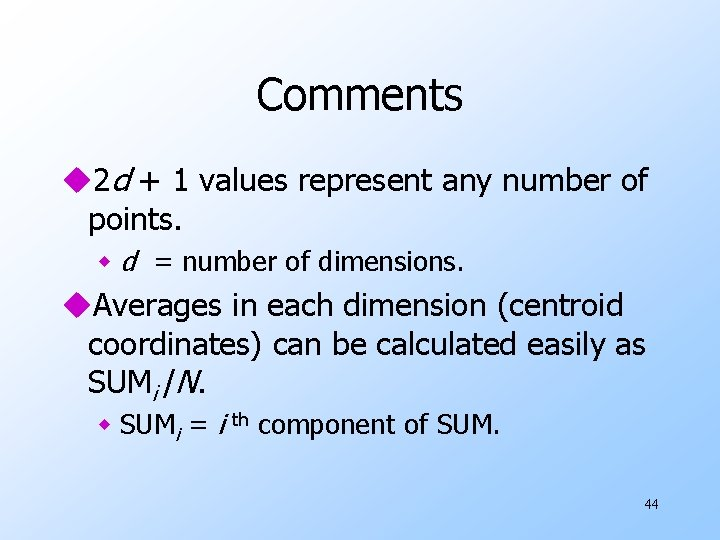Comments u 2 d + 1 values represent any number of points. w d = number of dimensions. u. Averages in each dimension (centroid coordinates) can be calculated easily as SUMi /N. w SUMi = i th component of SUM. 44Comments --- (2) u. Variance of a cluster’s discard set in dimension i can be computed by: SUMSQi /N – (SUMi /N )2 u. And the standard deviation is the square root of that. u. The same statistics can represent any compression set. 45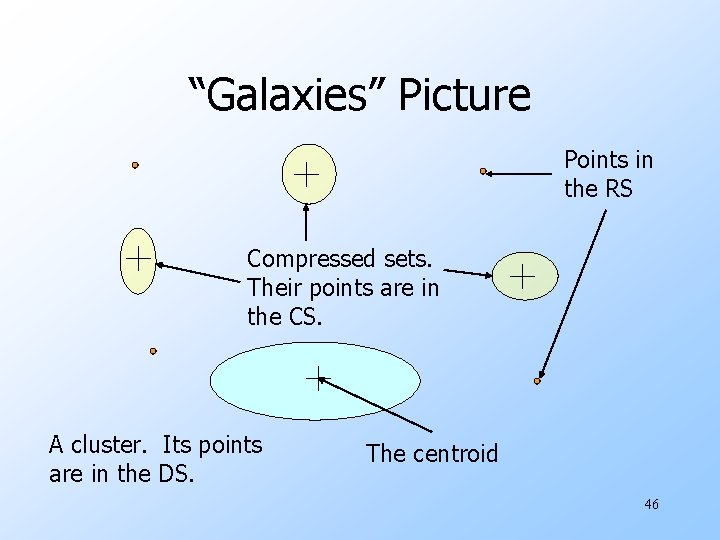“Galaxies” Picture Points in the RS Compressed sets. Their points are in the CS. A cluster. Its points are in the DS. The centroid 46Processing a Set of Points 1. Find those points that are “sufficiently close” to a cluster centroid, and add those points to the cluster and the DS. 2. Use any main-memory clustering algorithm to cluster the remaining points and the old RS. u Clusters go to the CS; outlying points to the RS. 47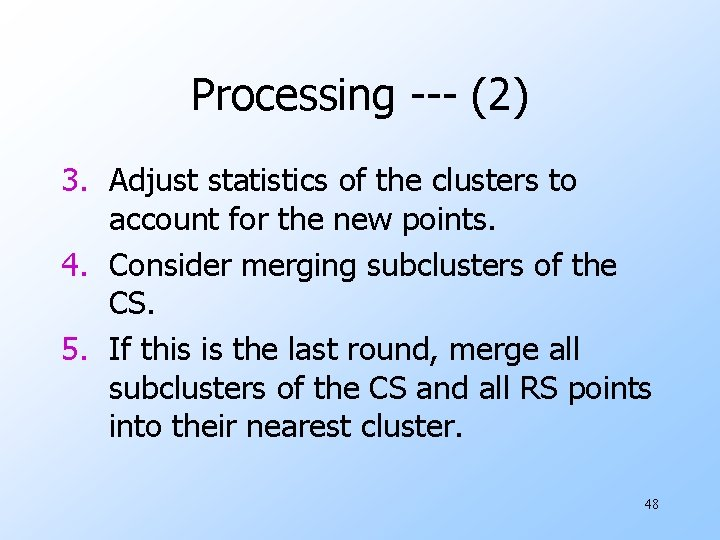Processing --- (2) 3. Adjust statistics of the clusters to account for the new points. 4. Consider merging subclusters of the CS. 5. If this is the last round, merge all subclusters of the CS and all RS points into their nearest cluster. 48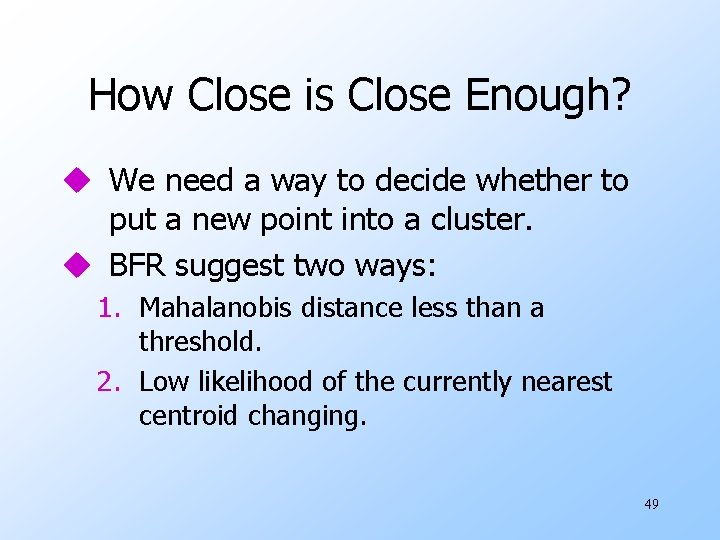How Close is Close Enough? u We need a way to decide whether to put a new point into a cluster. u BFR suggest two ways: 1. Mahalanobis distance less than a threshold. 2. Low likelihood of the currently nearest centroid changing. 49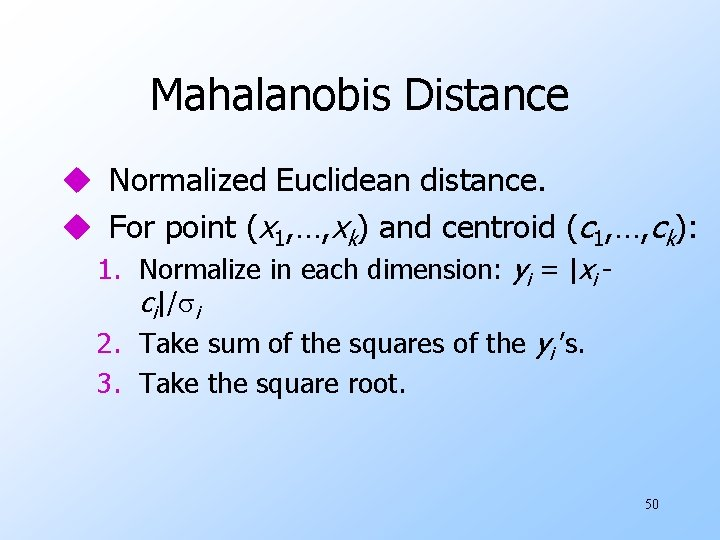Mahalanobis Distance u Normalized Euclidean distance. u For point (x 1, …, xk) and centroid (c 1, …, ck): 1. Normalize in each dimension: yi = |xi ci|/ i 2. Take sum of the squares of the yi ’s. 3. Take the square root. 50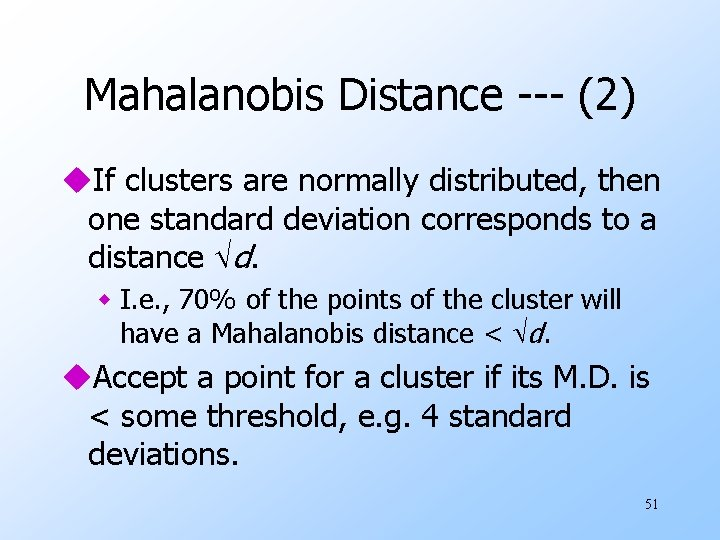Mahalanobis Distance --- (2) u. If clusters are normally distributed, then one standard deviation corresponds to a distance d. w I. e. , 70% of the points of the cluster will have a Mahalanobis distance < d. u. Accept a point for a cluster if its M. D. is < some threshold, e. g. 4 standard deviations. 51Picture: Equal M. D. Regions 2 52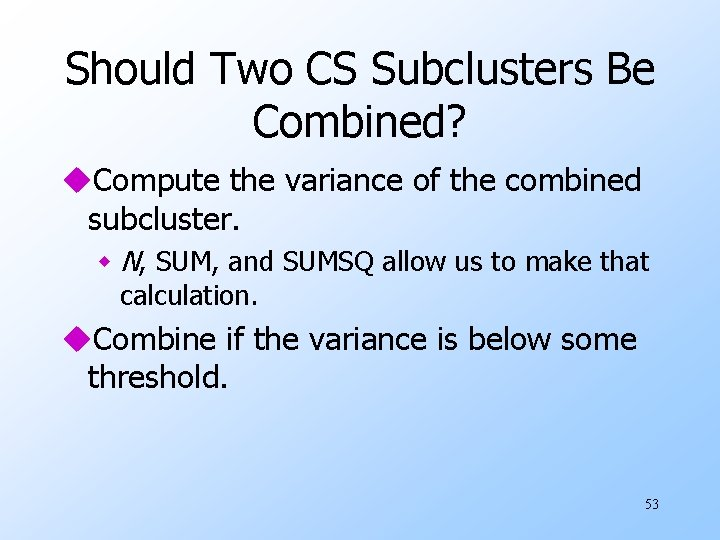Should Two CS Subclusters Be Combined? u. Compute the variance of the combined subcluster. w N, SUM, and SUMSQ allow us to make that calculation. u. Combine if the variance is below some threshold. 53